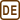## Radiation and current – voltage characteristic of a light bulb

In real life the current flowing through a light bulb is not proportional to the voltage (i.e. VA – characteristic of the light bulb is not linear) due to the current flow the tungsten filament starts to heat up and its resistivity starts to increase. If the temperature of the filament is greater than the ambient temperature we can assume:

• Its temperature is given by the equilibrium between the Joule heating caused by the flow of the electric current and the energy radiated to the surroundings. The tungsten filament can be considered as a black body.

• Electrical resistance of the bulb’s filament is proportional to the thermodynamic temperature.

Determine the dependence of the electric current flowing through the bulb on the connected voltage.

• #### Hint 1

The relation between the voltage U and the current I is described through the Ohm’s law

$I = \frac{U}{R}.$

We need to substitute the relation for resistance R which is proportional to thermodynamic temperature of the filament T, i.e.

$R=AT,$

where A is a constant.

Now we are left to determine the relation between thermodynamic temperature T and current and voltage.

• #### Hint 2

In a steady state, the power of the electric current Pel

$P_{el}=UI$

is equal to the radiated power Pr which is described by the Stefan-Boltzmann law for a black body

$P_r=\sigma ST^4,$

where σ = 5.67·10−8 Wm−2K−4 is the Stefan-Boltzmann constant and S is area.

From there we can determine the temperature of the filament.

• #### Analysis

If there weren’t a change in electric resistance of the bulb’s filament than the current would be proportional to the voltage. With increase of the current the bulb’s filament starts to heat up more and more and the electric resistance increases too. The current increases more slowly compared to the situation with constant resistance of the filament.

The electric current flowing through the bulb’s filament releases Joule heat that heats up the filament. The filament also radiates energy in the form of radiation to the surroundings. The amount of radiated energy is proportional to T 4 (with the assumption that the filament acts like a black body according to the Stefan-Boltzmann law) where T is the thermodynamic temperature. We can derive the formula for temperature with the relation to electric voltage and current. We will substitute this formula to the Ohm’s law.

• #### Solution

In a steady state, the power of electric current Pel is equal to the radiated power Pr.

The electric power is given by the multiplication of voltage U and current I:

$P_{el}=UI .$

Radiated power for the black body is described by the Stefan-Boltzmann law

$P_{r}=σST^{4} ,$

where σ = 5.67·10−8 Wm−2K−4 is the Stefan-Boltzmann constant and S is area. From there we can determine the temperature of the filament

$UI=\sigma ST^4$ $T=\sqrt{\frac{UI}{\sigma S}}.$

We need to determine the correlation between the current I and voltage U. That is given by Ohm’s law:

$I=\frac{U}{R},$

where electric resistance R is given to be proportional to the thermodynamic temperature (A is a proportionality constant): $$R=AT$$.

We will substitute the formula for resistance R and the thermodynamic temperature T into the Ohm’s law:

$I=\frac{U}{AT}=\frac{U}{A\sqrt\frac{UI}{\sigma S}}$

and derive the formula for the current I:

$I=\frac{U}{A}\sqrt{\frac{\sigma S}{UI}}\hspace{15px}/^4$ $I^4=\frac{U^4}{A^4}\,\frac{\sigma S}{UI}$ $I^5=\frac{\sigma S}{A^4}U^3\hspace{15px}/\sqrt{()}$ $I=\sqrt{\frac{\sigma S}{A^4}}\,U^{\frac{3}{5}}$ $I=CU^{\frac{3}{5}}.$

In the last relation we labelled the combination of the constants A, S and σ as C. This constant describes the characteristics of the light bulb. We can also notice that with increasing voltage the increase in current is slower than if these two would be proportional to each other from the last formula.

The current flowing through the light bulb is proportional to $$U^{\frac{3}{5}}$$ in case of much greater temperatures of the filament than the ambient temperature.